JMSLTM Numerical Library 7.2.0
com.imsl.stat

## Class WilcoxonRankSum

• All Implemented Interfaces:
Serializable, Cloneable

```public class WilcoxonRankSum
extends Object
implements Serializable, Cloneable```
Performs a Wilcoxon rank sum test.

Class `WilcoxonRankSum` performs the Wilcoxon rank sum test for identical population distribution functions. The Wilcoxon test and the Mann-Whitney U test are equivalent. If the difference between the two populations can be attributed solely to a difference in location, then the Wilcoxon test becomes a test of equality of the population means (or medians) and is the nonparametric equivalent of the two-sample t-test. Class `WilcoxonRankSum` obtains ranks in the combined sample after first eliminating missing values from the data. The rank sum statistic is then computed as the sum of the ranks in the `x` sample. Three methods for handling ties are used. (A tie is counted when two observations are within `fuzz` of each other.) Method 1 uses the largest possible rank for tied observations in the smallest sample, while Method 2 uses the smallest possible rank for these observations. Thus, the range of possible rank sums is obtained.

Method 3 uses the average rank of the tied observations for handling tied observations between samples. Asymptotic standard normal scores are computed for the W score (based on a variance that has been adjusted for ties) when average ranks are used (see Conover 1980, p. 217), and the probability associated with the two-sided alternative is computed.

The p-value returned in `stat` is the two-sided p-value calculated using the normal approximation with the normal score returned in `stat`.

Hypothesis Tests

In each of the following tests, the first line gives the hypothesis (and its alternative) under the assumptions 1 to 3 below, while the second line gives the hypothesis when assumption 4 is also true. The rejection region is the same for both hypotheses and is given in terms of Method 3 for handling ties. Another output statistic should be used, ( `stat` or `stat`, where `stat` is the array containing the statistics returned from the `getStatistics` method), if another method for handling ties is desired.

 Test Null Hypothesis Alternative Hypothesis Action 1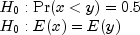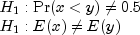Reject if `stat` is less than the significance level of the test. Alternatively, reject the null hypothesis if `stat` is too large or too small. 2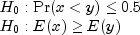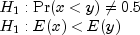Reject if `stat` is too small. 3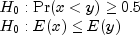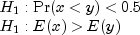Reject if `stat` is too large.

Assumptions

1. x and y contain random samples from their respective populations.
2. All observations are mutually independent.
3. The measurement scale is at least ordinal (i.e., an ordering less than, greater than, or equal to exists among the observations).
4. If f(x) and g(y) are the distribution functions of x and y, then g(y) = f(x + c) for some constant c(i.e., the distribution of y is, at worst, a translation of the distribution of x).

The p-values are calculated using either the large-sample normal approximation or the exact probability calculations. The approximate calculation returned by the `compute` method is usually considered adequate when the size of one or both samples is greater than 50. For smaller samples, the exact probability calculations returned by the `computeExactPValues` method are recommended.

Example 1, Example 2, Serialized Form
• ### Constructor Summary

Constructors
Constructor and Description
```WilcoxonRankSum(double[] x, double[] y)```
Constructor for `WilcoxonRankSum`.
• ### Method Summary

Methods
Modifier and Type Method and Description
`double` `compute()`
Performs a Wilcoxon rank sum test using an approximate p-value calculation.
`double[]` `computeExactPValues()`
Performs a Wilcoxon rank sum test using exact p-value calculations.
`double` `getMannWhitney()`
Returns the Mann-Whitney test statistic.
`int` `getNumberMissingX()`
Returns the number of missing observations detected in `x`.
`int` `getNumberMissingY()`
Returns the number of missing observations detected in `y`.
`double[]` `getStatistics()`
Returns the statistics.
`void` `setFuzz(double fuzz)`
Sets the nonnegative constant used to determine ties in computing ranks in the combined samples.
• ### Methods inherited from class java.lang.Object

`clone, equals, finalize, getClass, hashCode, notify, notifyAll, toString, wait, wait, wait`
• ### Constructor Detail

• #### WilcoxonRankSum

```public WilcoxonRankSum(double[] x,
double[] y)```
Constructor for `WilcoxonRankSum`.
Parameters:
`x` - A `double` array containing the first sample.
`y` - A `double` array containing the second sample.
• ### Method Detail

• #### compute

`public final double compute()`
Performs a Wilcoxon rank sum test using an approximate p-value calculation.
Returns:
A `double` scalar containing the two-sided p-value for the Wilcoxon rank sum statistic that is computed with average ranks used in the case of ties.
• #### computeExactPValues

`public final double[] computeExactPValues()`
Performs a Wilcoxon rank sum test using exact p-value calculations.
Returns:
A `double` array containing the the exact p-values according to the following table:  Row p-values 0 The exact left-tailed p-value. 1 The exact right-tailed p-value. 2 The exact two-tailed p-value.

• #### getMannWhitney

`public double getMannWhitney()`
Returns the Mann-Whitney test statistic.
Returns:
A `double` scalar containing the Mann-Whitney test statistic equivalent to the W statistic with average ranks used in case of ties. Although the test statistics for the Mann-Whitney and Wilcoxon rank sum tests are computed differently, the p-values for these tests are equal since the Wilcoxon test statistic is a linear transformation of the Mann-Whitney test statistic.
• #### getNumberMissingX

`public int getNumberMissingX()`
Returns the number of missing observations detected in `x`.
Returns:
An `int` scalar containing the number of missing observations in `x`.
• #### getNumberMissingY

`public int getNumberMissingY()`
Returns the number of missing observations detected in `y`.
Returns:
An `int` scalar containing the number of missing observations in `y`.
• #### getStatistics

`public double[] getStatistics()`
Returns the statistics. Note that the `compute` method must be invoked first before invoking this method. Otherwise, the method throws a `NullPointerException` exception.
Returns:
A `double` array of length 10 containing the following statistics:  Row Statistics 0 Wilcoxon W statistic (the sum of the ranks of the x observations) adjusted for ties in such a manner that W is as small as possible. 1 2 x E(W) - W, where E(W) is the expected value of W. 2 Probability of obtaining a statistic less than or equal to min{W, 2 x E(W) - W}. 3 W statistic adjusted for ties in such a manner that W is as large as possible. 4 2 x E(W) - W, where E(W) is the expected value of W, adjusted for ties in such a manner that W is as large as possible. 5 Probability of obtaining a statistic less than or equal to min{W, 2 x E(W) - W}, adjusted for ties in such a manner that W is as large as possible. 6 W statistic with average ranks used in case of ties. 7 Estimated standard error of Row 6 under the null hypothesis of no difference. 8 Standard normal score associated with Row 6. 9 Two-sided p-value associated with Row 8.

• #### setFuzz

`public void setFuzz(double fuzz)`
Sets the nonnegative constant used to determine ties in computing ranks in the combined samples.
Parameters:
`fuzz` - A `double` scalar containing the nonnegative constant used to determine ties in computing ranks in the combined samples. A tie is declared when two observations in the combined sample are within `fuzz` of each other. Default: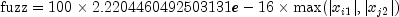JMSLTM Numerical Library 7.2.0